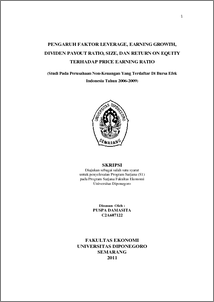# PENGARUH FAKTOR LEVERAGE, EARNING GROWTH, DIVIDEN PAYOUT RATIO, SIZE, DAN RETURN ON EQUITY TERHADAP PRICE EARNING RATIO (Studi Pada Perusahaan Non-Keuangan Yang Terdaftar Di Bursa Efek Indonesia Tahun 2006-2009)

DAMASITA, Puspa and WIDYARTI , Endang Tri (2011) PENGARUH FAKTOR LEVERAGE, EARNING GROWTH, DIVIDEN PAYOUT RATIO, SIZE, DAN RETURN ON EQUITY TERHADAP PRICE EARNING RATIO (Studi Pada Perusahaan Non-Keuangan Yang Terdaftar Di Bursa Efek Indonesia Tahun 2006-2009). Undergraduate thesis, Universitas Diponegoro.Preview
PDF - Published Version
1029Kb

## Abstract

The stock market is an indicator of the progress of a country's economy and to support economic development the country concerned. Various role of capital markets in a country that is as a facility to do the interaction between buyer and seller to determine the stock price. one analysis tool in the assessment of the stock price is Price Earning Ratio (PER). This study aims to examine the factors Leverage, Earning Growth, Dividend Payout Ratio (DPR), Size, and Return on Equity (ROE) of Price Earning Ratio (PER) on non-financial companies listed in Indonesia Stock Exchange for 2006 - 2009. This study used the entire population of non-financial companies listed in Indonesia Stock Exchange (BEI) in 2006 until 2009, a total of 283 companies. Sampling was conducted with a purposive sampling technique. With the sampling method used the samples obtained in this study as many as 14 companies. This study uses secondary data obtained from the publication of Indonesian Capital Market Directory (ICMD). The analysis technique used in this study is the technique of multiple regression analysis with least square equations and test the hypothesis F-statistic to test the effect together with the 5% confidence level and using the t-statistic for testing the partial regression coefficient. In the classical assumption test results showed that there were no deviations classical assumption, this shows that the available data has been qualified to use multiple linier regression model. From this reseach the following conclusion : the leverage variable is negative and significant effect on PER, variabel earning growth has positive and significant effect on PER, DPR variable has positive and significant effect on PER, Size variable has positive and not significant effect on PER, and ROE variable has positive and not significant on PER. Regression equation obtained is PER = 0,053 – 0,111 DER + 0,115 EG + 0,144 DPR + 0,728 SIZE + 0,007ROE. Coefficient of determination (adjusted 𝑅2) is 51,3% which means 51,3% of PER is influenced by the independent variables, whereas the remaining balance of 48,7% is explained by other variable not prensented in the study.

Item Type: Thesis (Undergraduate) Price Earning Ratio (PER), Leverage, Earning Growth, Dividen Payout Ratio (DPR), Size, Return on Equity (ROE) Price Earning Ratio (PER), Leverage, Earning Growth, Dividen Payout Ratio (DPR), Size, Return on Equity (ROE) H Social Sciences > H Social Sciences (General) Faculty of Economics and Business > Department of Management 28647 Mr. Perpustakaan Fakultas Ekonomi 18 Jul 2011 14:52 18 Jul 2011 14:52

Repository Staff Only: item control page